# What is Absolute reference in Excel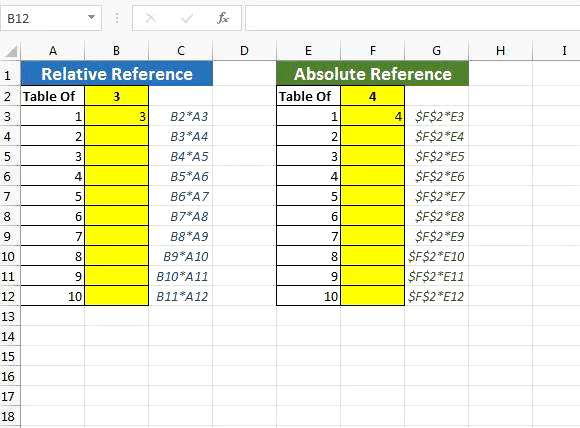What is an Absolute Reference in Excel

You must have read the word absolute reference. If not, you must have noticed the \$ (dollar) sign before cell references (\$A\$1). They are absolute references. When we prefix the \$ sign before a column letter and/or row number that row and/or column number becomes absolute.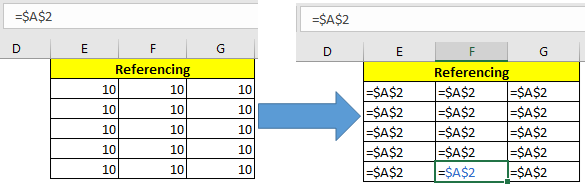What does excel absolute reference mean?

Well, when we don’t want cell references to change relatively while copying them in other cells, we use absolute references in excel. In other words, we lock or say freeze the cell or range using absolute referencing.

Generic Syntax of Absolute Referencing

Syntax to Freeze column only (Absolute Column Reference)

 =\$A1

Syntax to Freeze Row Only (Absolute Row Reference)

 =A\$1

Syntax of Freeze Cell (Absolute Cell Reference)

 =\$A\$1

Syntax of Expanding Range (Absolute Starting Cell Reference)

 =\$A\$1:A1

Syntax of Collapsing Range (Absolute Ending Cell Reference)

 =A1:\$A\$10

Syntax for Frozen Range (Absolute Starting Cell Reference)

 =\$A\$1:\$A\$10

Or toggle between absolute reference and relative reference

Use the F4 key on the window to toggle between Relative and Absolute References. Just select the range and press F4.If you press once: A1 - > \$A\$1

If you press twice: \$A\$1 -> A\$1

If you press thrice: A\$1 -> \$A1

If you press quad: \$A1 -> A1.

And it then it repeat this cycle

Example:

Excel Absolute Cell Reference

So to illustrate the use of all above types of Absolute referencing, I have this 2D matrix in excel.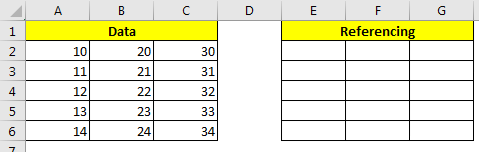In range A2:C6 I have some numbers. In range E2:G6 we will test above absolute referencing techniques of excel.

The shortcut to make absolute references is F4 while selecting the range in formula.

Absolute Column Only (Freeze Column)

When we only want to freeze columns only, we put \$ sign before the column letter of the cell. This is also called mixed referencing; since rows are relative and columns are absolute.

Let’s see the effect of it.

In E2, write this formula and hit enter.

 =\$A2

Now you can see the value of A2 in E2. Copy E2 in the rest of the cells in range E2:G6. What do you see?

You can see that the only A columns data is being copied in all cells in E2:G6. When you copy down the rows are changing. When you copy towards adjacent right or left, column referencing is not changing. This is because we freeze (\$) on the column (A).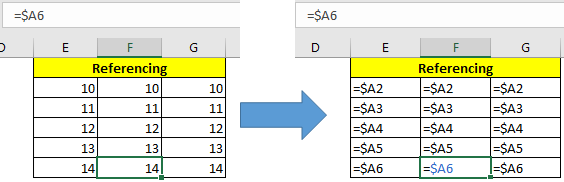Absolute Rows Only (Freeze Rows)

It is the same as freezing columns. We use \$ sign before row number and leave column number relative. This makes excel to freeze the row and keep columns relative.

Write this formula in E2 and copy E2 in rest of the range.

 =A\$2

You will see that, only row 2’s data is copied in all the rows since row 2 is frozen using \$ sign.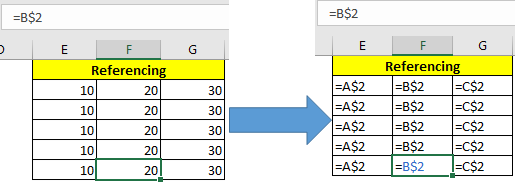Syntax of Freeze Cell Only (Absolute Cell Reference)

When we use \$ sign before both, column letter and row number, this become absolute cell reference in excel. It means when you copy formula, the formula will always refer to frozen cell.

Write this formula in E2 and copy E2 in rest of the range.

 =\$A\$2Expanding Range (Absolute Starting Cell Reference)

When we reference a range, and make the first cell absolute, this range becomes an expanding range. When we copy such formula, downwards or rightwards, the range expands.

Write this sum formula in E2 and copy E2 downwards and to the right of excel sheet.

 =SUM(\$A\$2:A2)

You’ll see that the first cell E2 has value A2 only. E3 cell has sum of A2:A3 and E6 has sum of A2:A6. Similar thing happens to the right of the sheet.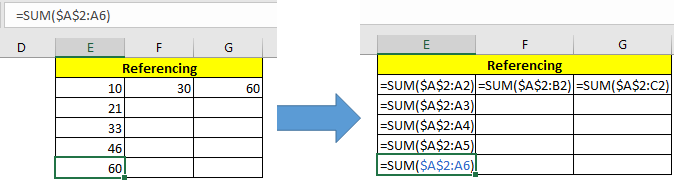Collapsing Range (Absolute Ending Cell Reference)

To the opposite of above technique, this type of reference collapses when you copy downwards.

Write this sum formula in E2 and copy E2 downwards.

 =SUM(A2:\$A\$6)

You can see that the first cell contains the sum of A2:A6, next A3:A6 and the last cell contains A6:A6.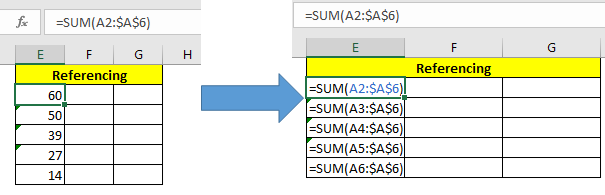Absolute Ranges in Excel

This is one of most used types of absolute referencing. Here we make the whole range absolute.

Write this formula in cell E2 and copy E2 in other cells.

 =SUM(\$A\$2:\$A\$6)

You can see that all the cells have a sum of A2:A6.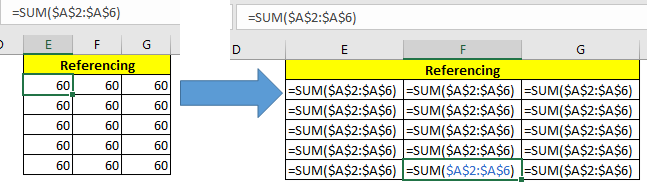Most of the people make mistakes while using lookup formulas by giving relative references of lookup range. You should always give absolute references of lookup range while using any lookup formula. This will make sure that lookup range doesn’t change (until you deliberately want it) while you copy formulas in other cells.

Eg =VLOOKUP (E2,\$A\$2:\$A\$6,1,0).

Example :

All of these might be confusing to understand. Let's understand how to use the function using an example. Here we will find the sum of the corresponding values.

Use the formula

 =SUM(D\$3,\$C4)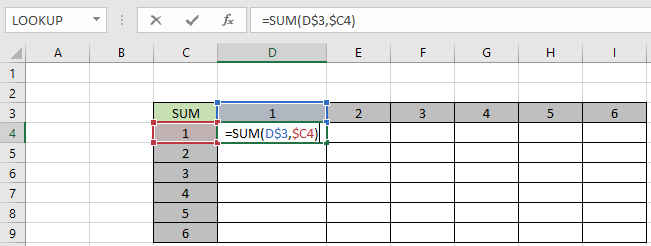Here we need to fix the D3 cell when copying and pasting the formula along the D column and fix the C4 cell when copying and pasting the formula along the row.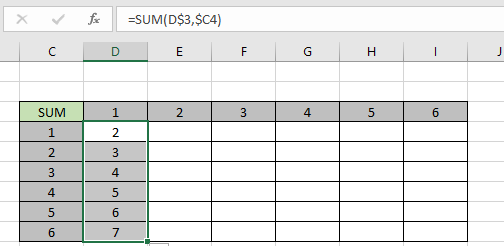As you can see the formula works fine when pasting formulas along the column. Now paste formula along the row to understand its feature.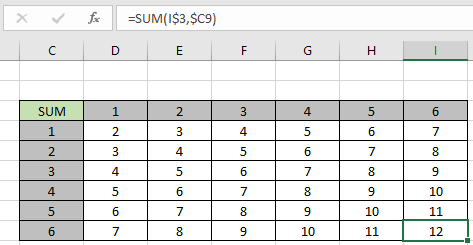As you can see, the formula works fine for both rows and columns. You can also copy (ctrl + C) and paste (Ctrl + V) to use the same feature.

Here are all the observational notes using the method in Excel
Notes :

1. Use Ctrl + D or drag down option to copy and paste the formula from top most cell to all the selected cells below
2. Use Ctrl + R or the drag right option to copy and paste the formula from the left most cell to all the selected cells on the right.
3. Or use the paste special method to learn more about copying and pasting values in Excel

Hope this article about What is Absolute reference in Excel is explanatory. Find more articles on referencing values and related Excel shortcuts here. If you liked our blogs, share it with your friends on Facebook. And also you can follow us on Twitter and Facebook. We would love to hear from you, do let us know how we can improve, complement or innovate our work and make it better for you. Write to us at info@exceltip.com.

Related Articles:

How to use the Shortcut To Toggle Between Absolute and Relative References in Excel : F4 shortcut to convert absolute to relative reference and same shortcut use for vice versa in Excel.

How to use Shortcut Keys for Merge and Center in Excel : Use Alt and then follow h, m and c to Merge and centre cells in Excel.

How to Select Entire Column and Row Using Keyboard Shortcuts in Excel : Use Ctrl + Space to select whole column and  Shift + Space to select whole row using keyboard shortcut in Excel

Paste Special Shortcut in Mac and Windows : In windows, the keyboard shortcut for paste special is Ctrl + Alt + V. Whereas in Mac, use Ctrl + COMMAND + V key combination to open the paste special dialog in Excel.

How to Insert Row Shortcut in Excel : Use Ctrl + Shift + = to open the Insert dialog box where you can insert row, column or cells in Excel.

Popular Articles :

How to use the IF Function in Excel : The IF statement in Excel checks the condition and returns a specific value if the condition is TRUE or returns another specific value if FALSE.

How to use the VLOOKUP Function in Excel : This is one of the most used and popular functions of excel that is used to lookup value from different ranges and sheets.

How to use the SUMIF Function in Excel : This is another dashboard essential function. This helps you sum up values on specific conditions.

How to use the COUNTIF Function in Excel : Count values with conditions using this amazing function. You don't need to filter your data to count specific values. Countif function is essential to prepare your dashboard.

Terms and Conditions of use

The applications/code on this site are distributed as is and without warranties or liability. In no event shall the owner of the copyrights, or the authors of the applications/code be liable for any loss of profit, any problems or any damage resulting from the use or evaluation of the applications/code.